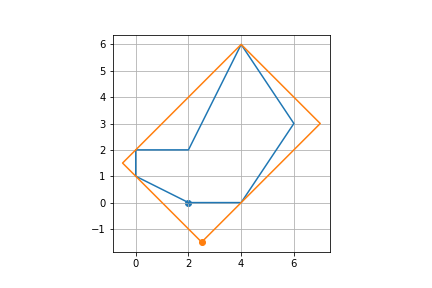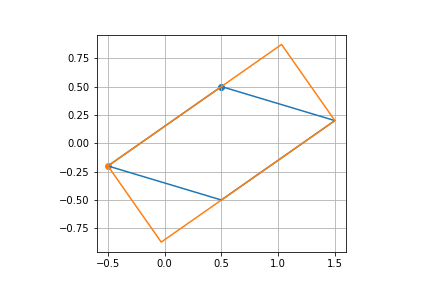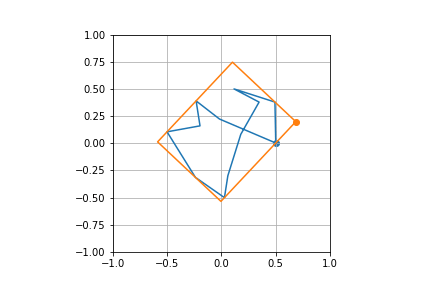{{ message }}

Instantly share code, notes, and snippets.

# janhuenermann/min-rotated-rect.py

Last active May 21, 2021
Find the smallest rotated rectangle that covers a given polygon
This file contains bidirectional Unicode text that may be interpreted or compiled differently than what appears below. To review, open the file in an editor that reveals hidden Unicode characters. Learn more about bidirectional Unicode characters
 import numpy as np # Gift wrapping algorithm, from https://en.wikipedia.org/wiki/Gift_wrapping_algorithm def find_convex_hull(poly): """ Returns the convex hull of the given polygon Can be replaced using OpenCV: ``` hull = cv2.convexHull(poly, clockwise=True, returnPoints=False) return poly[hull[:, 0]] ``` """ n = len(poly) p_i = p_0 = np.argmin(poly[:, 0]) hull = [] while p_i != p_0 or len(hull) == 0: hull.append(p_i) p_e = 0 # For each vertex, test if p_j is left of line between p_i <-> p_e for p_j in range(n): if p_e == p_i or np.cross(poly[p_e], poly[p_j] - poly[p_i]) > np.cross(poly[p_i], poly[p_j]): p_e = p_j p_i = p_e return np.array([poly[k] for k in hull]) def find_min_rotated_rect(poly): """Returns the four points of the smallest rotated rect that covers the given poly""" ch = find_convex_hull(poly) edges = ch - np.roll(ch, 1, 0) edges = edges / np.linalg.norm(edges, 2, -1)[:, None] normals = np.stack((-edges[:, 1], edges[:, 0]), 1) basis = np.stack((edges, normals), -1) ps = np.matmul(ch, basis) ps0 = np.amin(ps, 1) ps1 = np.amax(ps, 1) areas = np.prod(ps1 - ps0, -1) k = np.argmin(areas) rotated_rect = np.array( [[ps0[k, 0], ps0[k, 1]], [ps0[k, 0], ps1[k, 1]], [ps1[k, 0], ps1[k, 1]], [ps1[k, 0], ps0[k, 1]]]) return rotated_rect @ basis[k].T

### janhuenermann commented May 19, 2021 • edited

Test cases

```from matplotlib import pyplot as plt

# Only for visualisation
def plot_poly(poly):
poly = np.concatenate([poly, poly[0:1]], 0)
plt.plot(poly[:, 0], poly[:, 1])```
```# Test case 1
poly = np.array([[0., 1.], [0., 2.], [2., 2.], [4., 6.], [6., 3.], [4., 0.], [2., 0.]])
poly = np.roll(poly, 1, 0)
rect = find_min_rotated_rect(poly)
rect = norm_rect_with_ll_point(rect, poly)

plot_poly(poly)
plot_poly(rect)

plt.scatter(poly[0, 0], poly[0, 1])
plt.scatter(rect[0, 0], rect[0, 1])

plt.axis('scaled')
plt.grid('on')
plt.show()``````# Test case 2
poly = np.array([[0.5, 0.5], [-0.5, -0.2], [0.5, -0.5], [1.5, 0.2], [0.5, 0.5]])
rect = find_min_rotated_rect(poly)
rect = norm_rect_with_ll_point(rect, poly)

plot_poly(poly)
plot_poly(rect)

plt.scatter(poly[0, 0], poly[0, 1])
plt.scatter(rect[0, 0], rect[0, 1])

plt.axis('scaled')
plt.grid('on')
plt.show()```### janhuenermann commented May 19, 2021 • edited

Here's another test case

```poly = np.zeros((n, 2))
for i in range(1, n):
poly[i] = poly[i-1] + np.random.normal(size=(2,)) * 0.33

poly[:, 0] = 1. * (poly[:, 0] - np.min(poly[:, 0])) / (np.max(poly[:, 0]) - np.min(poly[:, 0])) - .5
poly[:, 1] = 1. * (poly[:, 1] - np.min(poly[:, 1])) / (np.max(poly[:, 1]) - np.min(poly[:, 1])) - .5

rect = find_min_rotated_rect(poly)
rect = norm_rect_with_ll_point(rect, poly)

plot_poly(poly)
plot_poly(rect)

plt.xlim(-1., 1.)
plt.ylim(-1., 1.)

plt.scatter(poly[0, 0], poly[0, 1])
plt.scatter(rect[0, 0], rect[0, 1])

plt.grid('on')
plt.show()```

And an animated version of the outputs:to join this conversation on GitHub. Already have an account? Sign in to comment